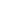Flat 50% off

Ends in

• JEE Main & Advanced
•• Mathematics
• Back to Doubt Clearing

## The complete set of the positive real values of the parameter 'a' for which the equation $|sin 2x| - |x| - a = 0$ does not have any real solution, is(a) $\left(\frac{3 \sqrt{3}+\pi}{6}, \infty\right)$(b) $\left(\frac{3 \sqrt{3}-\pi}{6}, \infty\right)$(c) $\left(\frac{3 \sqrt{3}-\pi}{12}, \infty\right)$(d) $\left(\frac{3 \sqrt{3}+\pi}{12}, \infty\right)$

### Asked By sai krishna

Updated Wed, 22 May 2019 05:29 pm